# Algebra II : Factorials

## Example Questions

1 2 3 4 5 6 7 8 10 Next →

### Example Question #91 : Factorials

Multiply: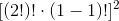Explanation:

Simplify all the terms in the parentheses first.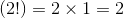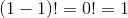This indicates that: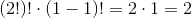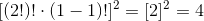The answer is:### Example Question #92 : Factorials

Evaluate: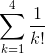None of the other choices gives the correct response.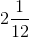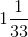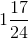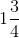Explanation:is equal to the sum of the expressions formed by substituting 1, 2, 3 and 4, in turn, forin the expression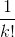, as follows: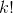- orfactorial - is defined to be the product of the integers from 1 to. Therefore, each term can be calculated by multiplying the integers from 1 to, then taking the reciprocal of the result.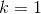: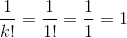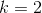: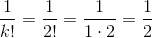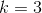: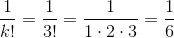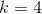: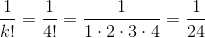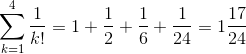### Example Question #93 : Factorials

Try without a calculator:

True or false: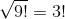False

True

False

Explanation: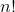- orfactorial - is defined to be the product of the integers from 1 to. Therefore,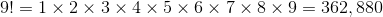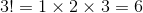Since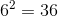,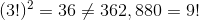andis a false statement.

### Example Question #94 : Factorials

Try without a calculator.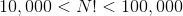Give the value ofthat makes this three-part inequality true.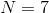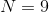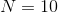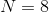The inequality has no solution.Explanation:

Given a nonnegative integer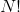- orfactorial - is defined to be the product of the integers from 1 to. After some exploration, multiplying 1 by 2, the result by 3, that result by 4, and so forth, we find that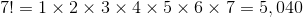Multiplying by 8, we find that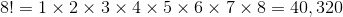Multiplying by 9, we find thatTherefore,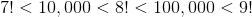Since only integers may have factorial values, it follows that the only value ofthat makes the inequality true is.

### Example Question #95 : Factorials

Try without a calculator: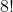is equal to which expression?

None of these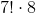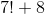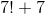Explanation:- orfactorial - is defined to be the product of the integers from 1 to. Therefore,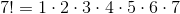and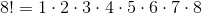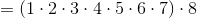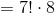,

the correct response.

### Example Question #96 : Factorials

Try without a calculator:

Which is true about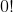?

Cannot be determinedis an undefined quantity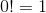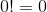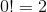Explanation:is considered to be equal to one by definition.

### Example Question #97 : Factorials

Try without a calculator:

Calculate: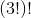Cannot be determined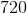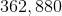Explanation:- orfactorial - is defined to be the product of the integers from 1 to. Therefore,Therefore,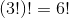Since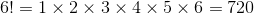,

it follows that 720 is the correct response.

1 2 3 4 5 6 7 8 10 Next →

### All Algebra II Resources